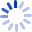# Schaum's Outline of Probability and Statistics

Helps to master probability and statistics. This guide includes chapters such as: Basic Probability; Random Variables and Probability Distributions; Mathematical Expectation; Special Probability Distributions; Sampling Theory; Estimation Theory; Tests of Hypotheses and Significance; and, Curve Fitting, Regression, and Correlation.

##### Cash for Textbooks